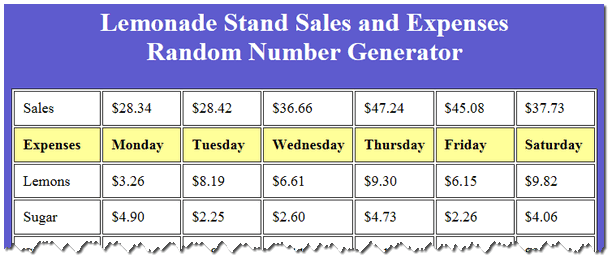×

# Excel Lesson Plan Lemonade Stand Expenses

Assignment: Students create an expense budget for a lemonade stand with 6 days of expenses.Students use the random number generator to get their item expenses for each day. Students enter their data in the spreadsheet for each day. Students use a formula (sum) to calculate the total expenses for each day.

## Random Number Generator Lemonade Stand Sales and Expenses

Students can use this online random number generator to help them create the numeric values for their spreadsheet. Simply click the "Get Numbers Now!" button to generate random sales and expense numbers for the lemonade stand.## Excel - Lemonade Stand Student Instructions

### Excel Mini-Course in 4 Minutes:Quickly learn the essentials you need for this lesson with these short, focused video tutorials. Watch all the videos to see how to create a budget and chart from start to finish or just watch the ones you need.

## Students Learn and Practice the Following Basic Spreadsheet Skills:

• Creating and formatting a spreadsheet title using "merge and center"
• Entering data in columns and rows
• Using the simple formula "Autosum" to automatically calculate total expenses
• Formatting numbers as currency and adding \$ signs.
• Creating a chart using the expense data entered.
• Using different colors for chart segments to improve visual presentation
• Creating, sizing, and positioning the chart to fit on a single page with the data
• Searching, inserting, sizing, and positioning clipart or pictures
• Using print preview and printing